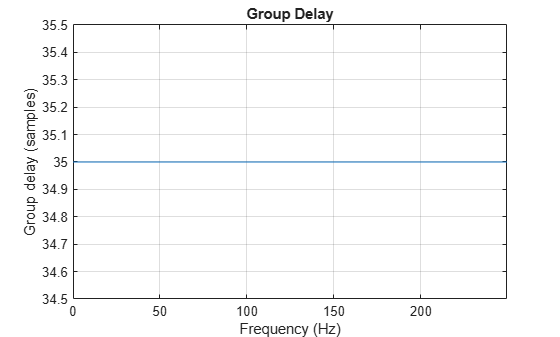# Compensate for Delay and Distortion Introduced by Filters

Filtering a signal introduces a delay. This means that the output signal is shifted in time with respect to the input.

When the shift is constant, you can correct for the delay by shifting the signal in time.

Sometimes the filter delays some frequency components more than others. This phenomenon is called phase distortion. To compensate for this effect, you can perform zero-phase filtering using the `filtfilt` function.

Take an electrocardiogram reading sampled at 500 Hz for 1 s. Add random noise. Reset the random number generator for reproducible results

```Fs = 500; N = 500; rng default xn = ecg(N)+0.1*randn([1 N]); tn = (0:N-1)/Fs;```

Remove some of the noise using a filter that stops frequencies above 75 Hz. Use `designfilt` to design an FIR filter of order 70.

```Nfir = 70; Fst = 75; firf = designfilt('lowpassfir','FilterOrder',Nfir, ... 'CutoffFrequency',Fst,'SampleRate',Fs);```

Filter the signal and plot it. The result is smoother than the original, but lags behind it.

```xf = filter(firf,xn); plot(tn,xn,tn,xf) title 'Electrocardiogram' xlabel 'Time (s)' legend('Original','FIR Filtered') grid```Use `grpdelay` to check that the delay caused by the filter equals half the filter order.

`grpdelay(firf,N,Fs)``delay = mean(grpdelay(firf))`
```delay = 35 ```

Line up the data. Shift the filtered signal by removing its first `delay` samples. Remove the last `delay` samples of the original and of the time vector.

```tt = tn(1:end-delay); sn = xn(1:end-delay); sf = xf; sf(1:delay) = [];```

Plot the signals and verify that they are aligned.

```plot(tt,sn,tt,sf) title 'Electrocardiogram' xlabel('Time (s)') legend('Original Signal','Filtered Shifted Signal') grid```Repeat the computation using a 7th-order IIR filter.

```Niir = 7; iir = designfilt('lowpassiir','FilterOrder',Niir, ... 'HalfPowerFrequency',Fst,'SampleRate',Fs);```

Filter the signal. The filtered signal is cleaner than the original, but lags in time with respect to it. It is also distorted due to the nonlinear phase of the filter.

```xfilter = filter(iir,xn); plot(tn,xn,tn,xfilter) title 'Electrocardiogram' xlabel 'Time (s)' legend('Original','Filtered') axis([0.25 0.55 -1 1.5]) grid```A look at the group delay introduced by the filter shows that the delay is frequency-dependent.

`grpdelay(iir,N,Fs)`Filter the signal using `filtfilt`. The delay and distortion have been effectively removed. Use `filtfilt` when it is critical to keep the phase information of a signal intact.

```xfiltfilt = filtfilt(iir,xn); plot(tn,xn) hold on plot(tn,xfilter) plot(tn,xfiltfilt) title 'Electrocardiogram' xlabel 'Time (s)' legend('Original','''filter''','''filtfilt''') axis([0.25 0.55 -1 1.5]) grid```